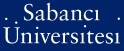# Bounded factorization property for Frechet spaces

Terzioğlu, Tosun and Zahariuta, Vyacheslav (2003) Bounded factorization property for Frechet spaces. Mathematische nachrichten, 253 (1). pp. 81-91. ISSN 0025-584XPDF - Registered users only - Requires a PDF viewer such as GSview, Xpdf or Adobe Acrobat Reader157Kb

Official URL: http://dx.doi.org/10.1002/mana.200310046

## Abstract

An operator T is an element of L(E, F) factors over G if T = RS for some S is an element of L(E, G) and R is an element of L(G, F); the set of such operators is denoted by L G (E, F). A triple (E, G, F) satisfies bounded factorization property (shortly, (E, G, F) is an element of BF) if L-G (E, F) subset of LB(E, F), where LB(E, F) is the set of all bounded linear operators from E to F. The relationship (E, G, F) is an element of BF is characterized in the spirit of Vogt's characterisation of the relationship L(E, F) = LB(E, F) . For triples of Kothe spaces the property BF is characterized in terms of their Kothe matrices. As an application we prove that in certain cases the relations L(E, G(1)) = LB(E, G(1)) and L(G(2), F) = LB(G(2), F) imply (E, G, F) is an element of BF where G is a tensor product of G(1) and G(2).

Item Type: Article Fréchet and Köthe spaces • continuous and bounded linear operators • projective tensor products Q Science > QA Mathematics 11446 Tosun Terzioğlu 10 Apr 2009 16:10 22 Jul 2019 15:35

Repository Staff Only: item control page